# Yield to Maturity (YTM)

It is the expected return an investor would earn if they hold the bond until its maturity.

It is the total return anticipated on a bond if it is held till maturity. The terms "book yield" and "redemption yield" are other terms for yield to maturity. Although it is expressed as an annual rate, yield to maturity is regarded as a long-term bond yield.It is, therefore, the internal rate of return (IRR) of a bond investment, assuming the investor retains the bond till maturity, with all scheduled payments made and reinvested at the same pace.

It is comparable to the current yield, which calculates how much money would be made by purchasing and holding a bond for a year by dividing annual cash inflows by its market price

However, YTM considers the present value of a bond's future coupon payments, unlike the current yield.

In other words, it takes into account the time value of money, whereas a straightforward computation of current yield does not. As a result, it is frequently seen as a more accurate way of determining a bond's return.
It can be quite helpful in deciding whether purchasing bonds is a wise investment. l. An investor needs to decide upon the required rate of return on the bond that will make the bond worthwhile.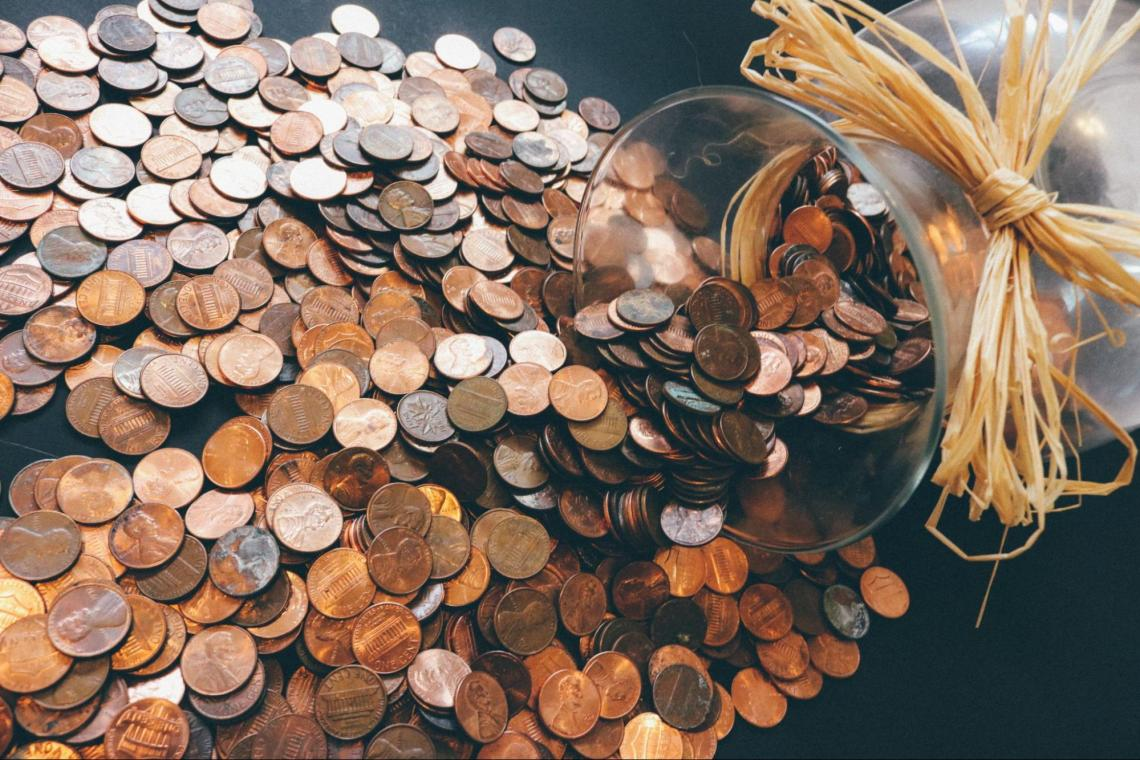An investor can decide whether a bond is a good investment by comparing the YTM to the required yield of a bond they are considering purchasing.

Since YTM expresses the value of multiple bonds in the same annual terms regardless of the bond's term to maturity, so it can be used to compare bonds with varying maturities and coupons.

Taxes that an investor pays on the bond are typically not taken into account in YTM calculations.

YTM, in this context, refers to the gross redemption yield. Additionally, buying and selling costs are not considered for YTM estimates.

YTM also assumes things about the future that are unknown now because the bond may not be kept until maturity; an investor might be unable to reinvest all of the coupons, or the bond's issuer might stop making payments.

## Types

There are multiple types of YTM. Their difference lies in the types of bonds they describe. A few types are listed below:

1. Zero-coupon bond YTM

The Yield to Maturity, a crucial indicator used in the bond market, expresses the overall rate of return anticipated from a bond once all of its future interest payments have been made, and its principal has been repaid.But unlike bonds with a coupon rate, zero-coupon bonds (z-bonds) don't have recurring interest payments, so their YTM calculations differ.

It is a significant factor since z-bonds are a prevalent type of debt issued by several entities, including some U.S. Treasury securities

These bonds are often issued at a discount in the market and subsequently mature to their face value rather than paying coupons.

The formula for finding the YTM for a zero-coupon bond is as follows: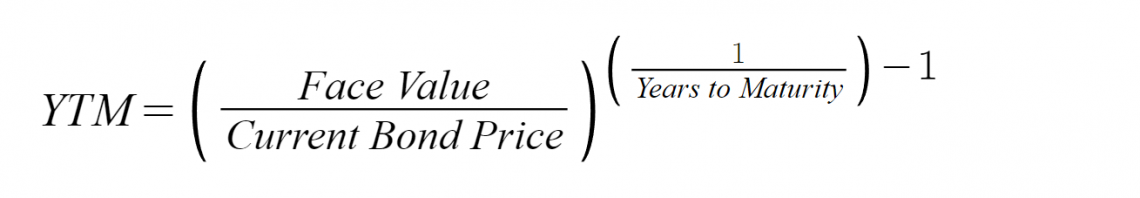For instance, if there is a \$1,000 zero-coupon bond that has two years until maturity. The bond is currently valued at a purchase price of \$915. By using the above formula, we can calculate the following: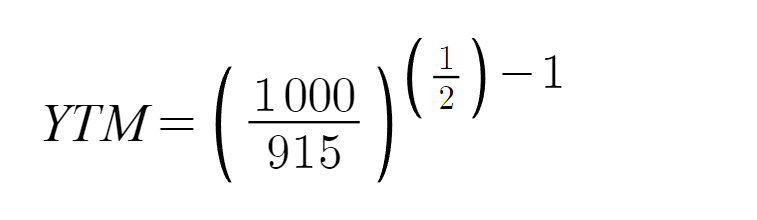The answer is 0.045, or 4.54%.

2. Estimated YTM

This type is widely used when calculating the YTM of long-term bond coupons.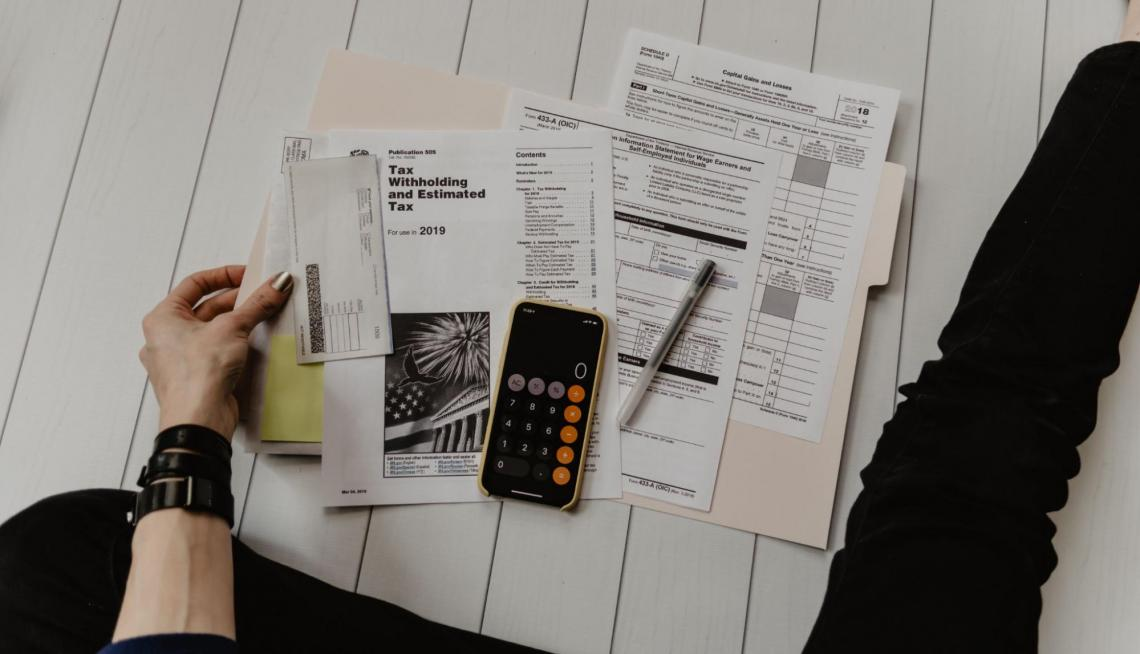However, the actual YTM must be determined through trial and error, taking into account various rates for the bond's current value until the price is close to the bond's actual market price.

These days, computer programs make it simple to calculate the bond's YTM. The yield to maturity is estimated using the formula below.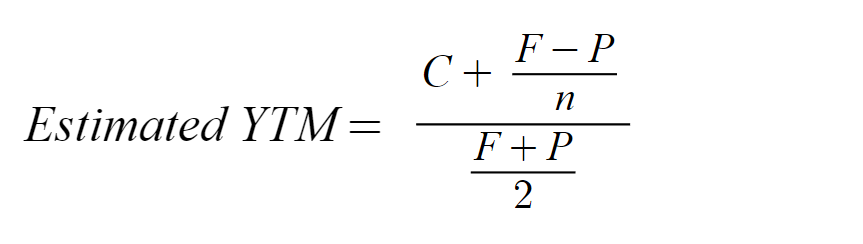Where,

• C = Coupon
• F = Face Value
• P = Price
• n = Years to maturity

For instance, if a bond costs \$920 and has a \$1,000 face value, which is usually the face value of many bonds. Assume that there are 10 years before maturity, the annual coupons are \$100, and the coupon rate is 10%. Using the formula, this illustration would be: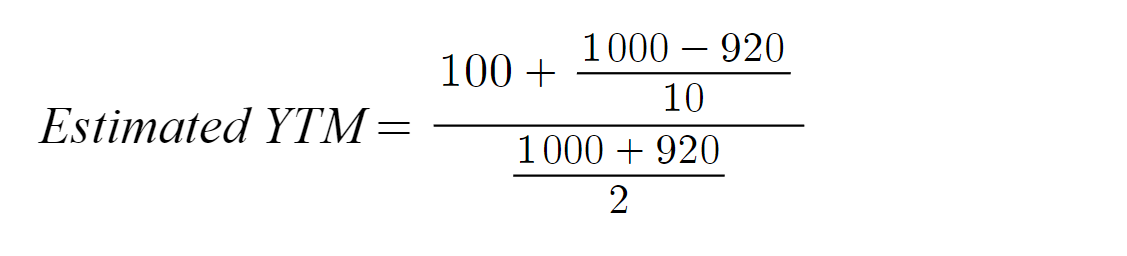Therefore, the estimated YTM on this bond is equal to 0.112 or 11.25%.

3. Coupon Bond YTM Trial and Error Method

There is one more way for investors to calculate the YTM of a coupon bond, including the one that uses its present value in its formula.

The present value of all future cash flows matches the bond's market price because YTM is the interest rate that an investor would receive if he or she reinvested each bond coupon payment at a constant interest rate until the bond's maturity date.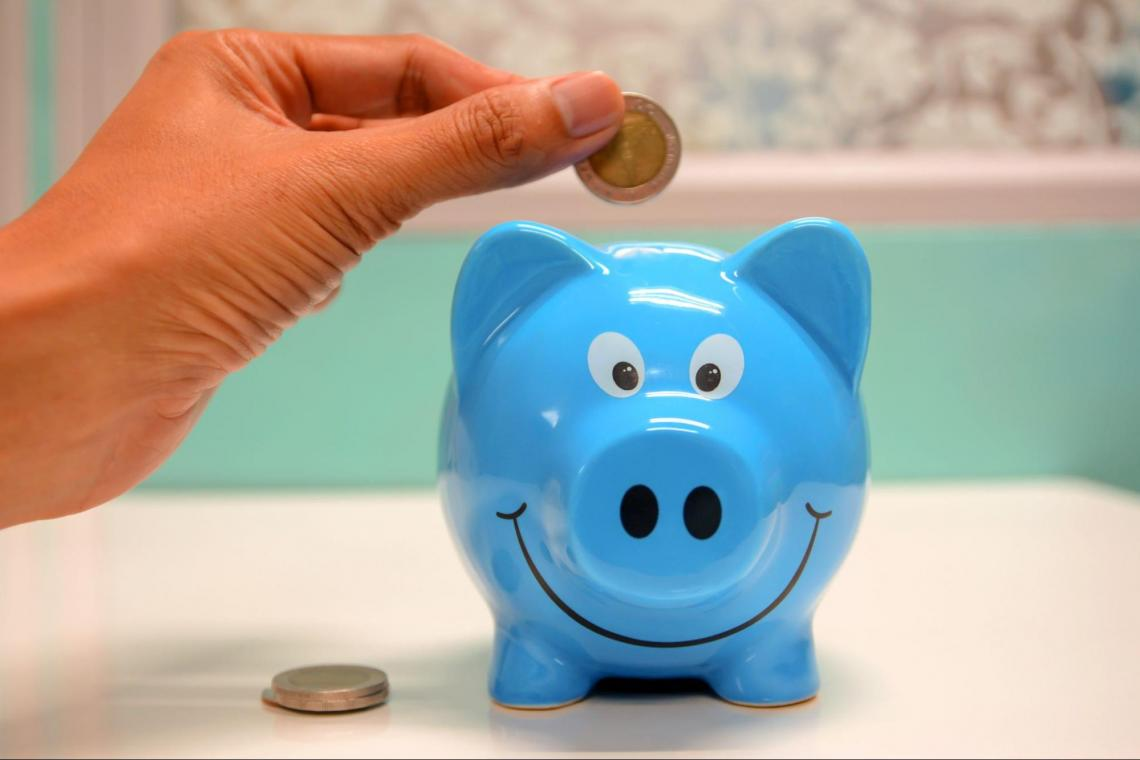Investors are aware of the bond's current price, coupon payments, and maturity amount but cannot determine the discount rate directly. However, using the subsequent present value calculation and trial-and-error, it is possible to determine YTM: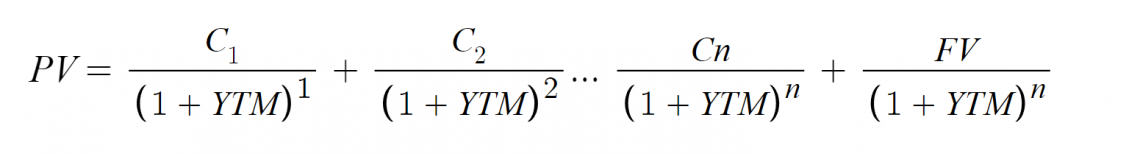YTM calculations consider the bond's current market price, par value, coupon interest rate, and term to maturity, as well as the assumption that all coupon payments will be reinvested at the same rate as the bond's current yield.

Coupon payments are not always investable at the same interest rate, so the YTM is only a snapshot of the return on a bond. Therefore, the YTM will grow as interest rates rise and fall as interest rates decline.

Determining an accurate YTM value is frequently challenging since yield to maturity determination is a complicated process. Instead, one can get a rough idea of YTM using a yield to maturity calculator, yield table, or financial calculator.

## YTM Variations

There are many variations of YTM. The difference between them lies in a specific bond's overall nature and options. Some of its variations are listed below:

1. Yield to Call (YTC)

YTC is a term used in finance to describe the return a bondholder will earn if they hold onto the bond until the call date, which happens before its maturity.

The yield to maturity (the entire return that will be paid out by a bond's expiration date) is often what a bond buyer pays attention to.

However, if a bond has the call option, bond buyers must also be aware of their return if the seller decides to utilize it.Callable bonds often have a higher yield to maturity because the issuer may "call" them if he.

This particular form of yield is mentioned when a bond has features that let the issuer close it out before it matures.

2. Yield to Worst

The "yield to worst" is the lowest yield that may be received on a bond that fully complies with the terms of its contract without defaulting.

This specific type of yield is relevant when the bond contains options that allow the issuer to close out the bond before it matures.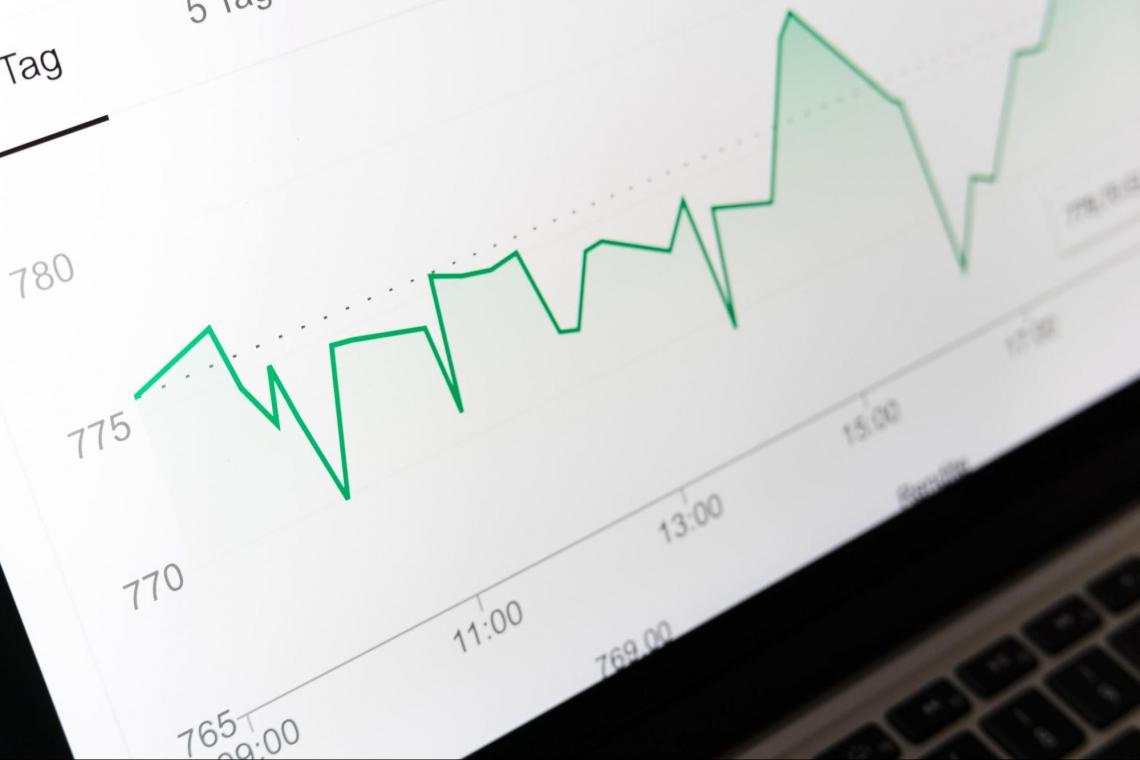A few clauses described in the bond's contract could be used to cause an early retirement of the bond, most commonly called callability.

The yield to worst metric was developed to evaluate the yield at the earliest possible retirement date under the identified worst-case scenario. YTW helps investors minimize risk and ensures that some income requirements will still be met even in the worst-case scenarios.

The yield to maturity of a bond is calculated using the earliest call or retirement date (YTM). It is assumed that a principal prepayment will occur if a bond issuer executes the call option.

Frequently, the principal is returned, and the call marks the end of the coupon payments. The issuer is likely to execute its callable option if yields are falling, and it can obtain a lower coupon rate through new issues, given the state of the market.

3. Yield to Put

Like, yield to call (YTC), yield to put (YTP) allows the holder of a put bond to decide whether to sell the bond back to the issuer at a set price per the bond's terms.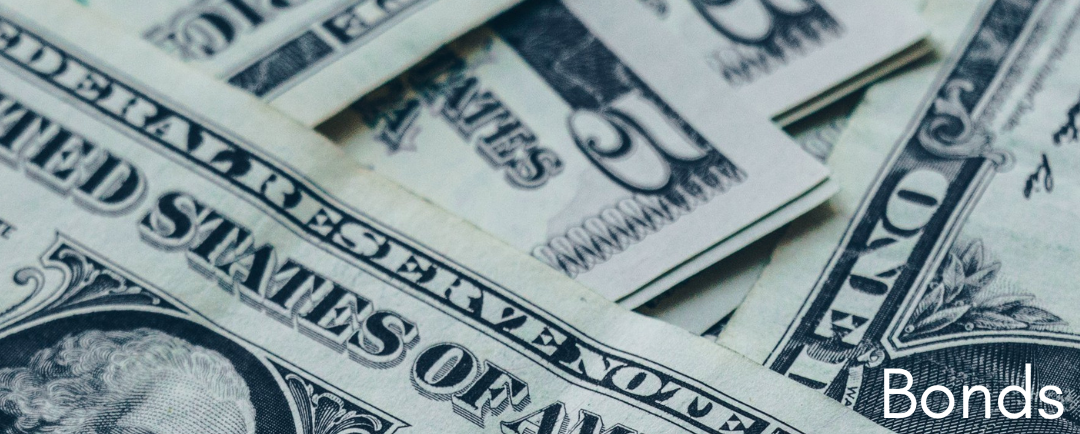The bond will be returned to the issuer as soon as it is practical and financially reasonable, according to the YTP calculation.

### Yield to Maturity vs. Coupon Rate

The YTM and the coupon rate are two critical factors that investors should examine when thinking about purchasing bonds.

Bonds of investment quality are low-risk investments that typically provide a return that is just a little bit higher than that of a typical savings account

Many investors consider these fixed-income investments as a reliable source of income in retirement. Any investor can add some bonds to their portfolio to reduce overall risk.The yield on a bond is defined as the effective rate of return based on the bond's actual market value. The coupon rate and yield are typically equal at face value when the bond is originally issued.

However, as buyers and sellers evaluate the yield offered in light of the changing interest rate environment, changes in interest rates will affect the bond's market value.

This creates an inverse relationship between the yield and the bond price, which causes both to move in opposite directions.

As a result, while the coupon rate for an earlier bond will remain the same, the bond's yield to maturity will change.

The number of income investors might anticipate receiving while holding the bond is known as the coupon rate or yield.

Although variable interest rates are possible for bonds, coupon rates are set when the government or company issues the bond.

These variable rate securities often use LIBOR or a similar generally accessible yield as a tying factor.

The coupon rate is the interest paid annually based on the financial instrument's face value or par value.

Some bonds might be set up to accrue interest over time. Additionally, there are specific dates for dividend payments (i.e., holders on the record date).

### FAQs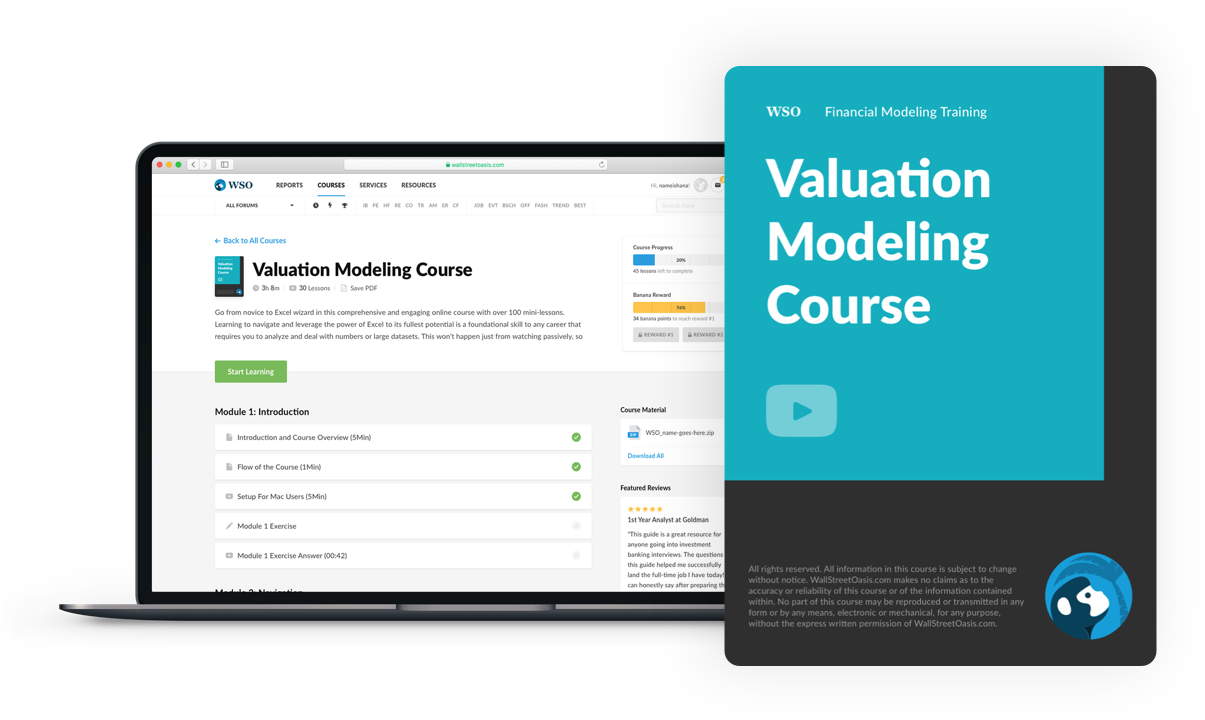### Everything You Need To Master Valuation Modeling

To Help You Thrive in the Most Prestigious Jobs on Wall Street.

Researched and authored by Anja Corbolokovic | Linkedin